# Om Prakash

•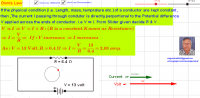### Ohm's Law (Explanation & Numerical)

Activity

Om Prakash

•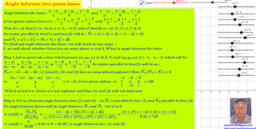### To derive Equation of Line and angle between two lines

Activity

Om Prakash

•### Derivation of Equation of Line in 3D geometry

Activity

Om Prakash

•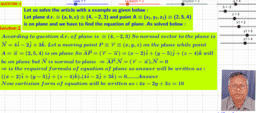### Method to find out vector & cartesian equation of plane in different cases.

Activity

Om Prakash

•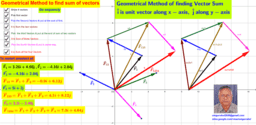### Vector sum of four forces (Vector Quantities )

Activity

Om Prakash

•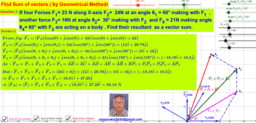### Vector Lesson-II (Geometrical Method of vector addition)

Activity

Om Prakash

•### Vector Lesson-1

Activity

Om Prakash

•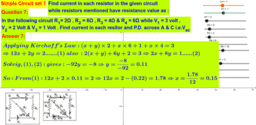### Numerical based on Kirchhoff's Law

Activity

Om Prakash

•### Quadratic Equation : Explanation & Solving Method

Activity

Om Prakash

•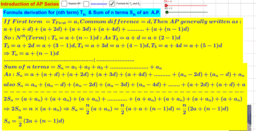### AP Series : Explaination & worked out Numericals

Activity

Om Prakash

•### Properties of Circle & it's proof

Activity

Om Prakash

•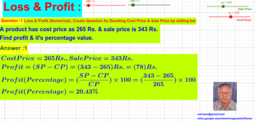### Loss & Profit : Numerical Practice

Activity

Om Prakash

•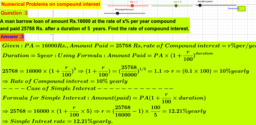### Compound & Simple Interest Calculation

Activity

Om Prakash

•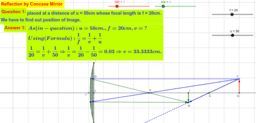### Reflection by Concave mirror explanation & Numerical Solving

Activity

Om Prakash

•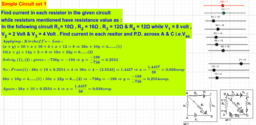### DC circuit solving by Kircoff's Law

Activity

Om Prakash

•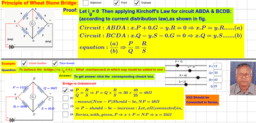### Wheatstone Bridge Numerical

Activity

Om Prakash

•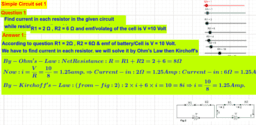### Numericals based on Kirchoff's Law

Activity

Om Prakash

•### Numerical on dot product,cross product ,dot-cross product of vectors

Activity

Om Prakash

•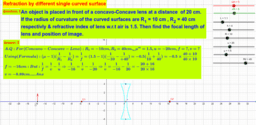### Refraction by Different Single Surface

Activity

Om Prakash

•### Refraction by different combination of curved surfaces

Activity

Om Prakash

•### Numerical based on different type of combination of curved surfaces of Refraction by

Activity

Om Prakash

•### Method to solve Numerical based on reflection by convex mirror

Activity

Om Prakash

•### Numerical Problem based on 'Reflection by Concave Mirror'

Activity

Om Prakash

•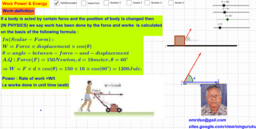Activity

Om Prakash